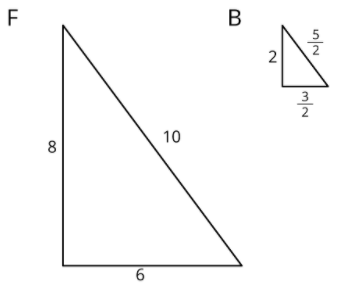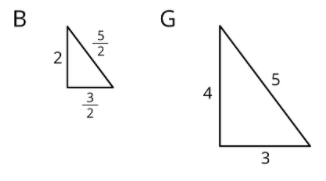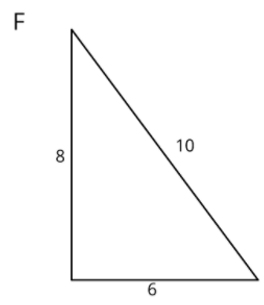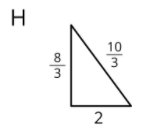# Scaled Copies

Find each of the following scale factors and explain how you know. The first one is done for you.
What is the scale factor that takes shape B to shape F? How do you know?The scale factor is 4, because 2 * 4 = 8, 3/2 * 4 = 6, and 5/2 * 4 = 10.

What is the scale factor that takes shape F to shape B? How do you know?What is the scale factor that takes shape B to shape G? How do you know?What is the scale factor that takes shape H to shape F? How do you know?